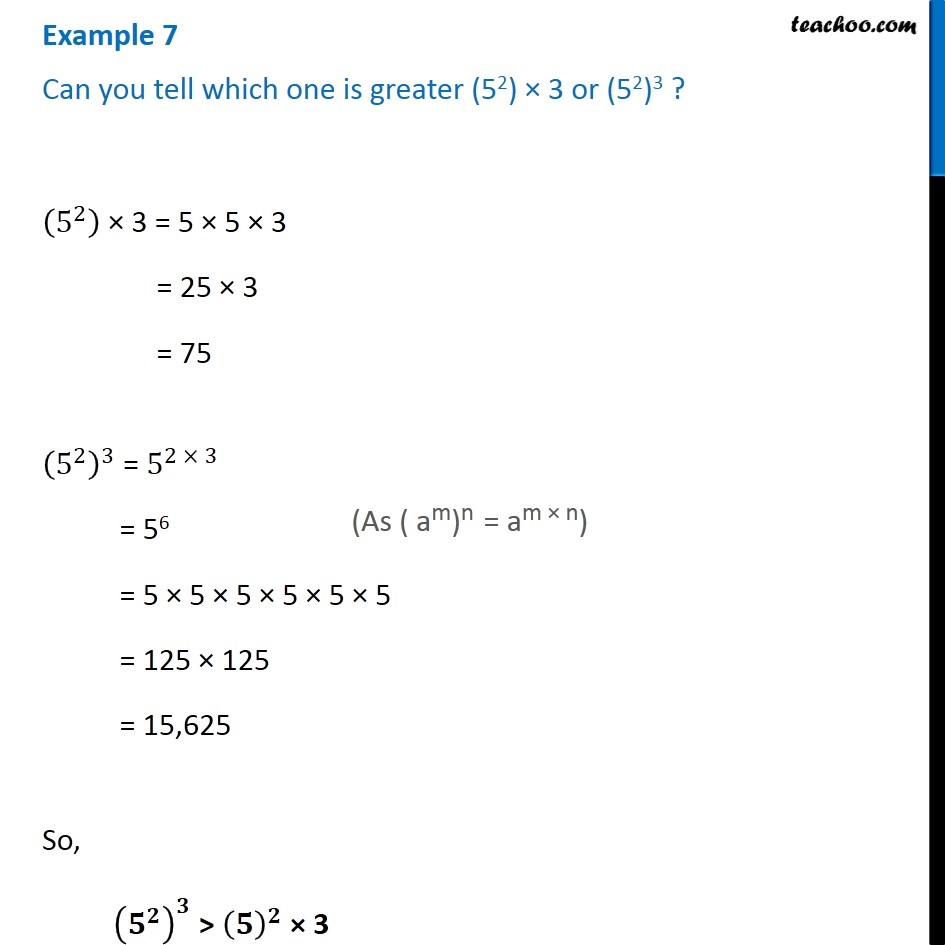1. Chapter 13 Class 7 Exponents and Powers
2. Concept wise
3. Law of exponents

Transcript

Example 7 Can you tell which one is greater (52) × 3 or (52)3 ? (5^2 ) × 3 = 5 × 5 × 3 = 25 × 3 = 75 (5^2 )^3 = 5^(2 × 3) = 56 = 5 × 5 × 5 × 5 × 5 × 5 = 125 × 125 = 15,625 So, (𝟓^𝟐 )^𝟑 > (𝟓)^𝟐 × 3 (As ( am)n = am × n)